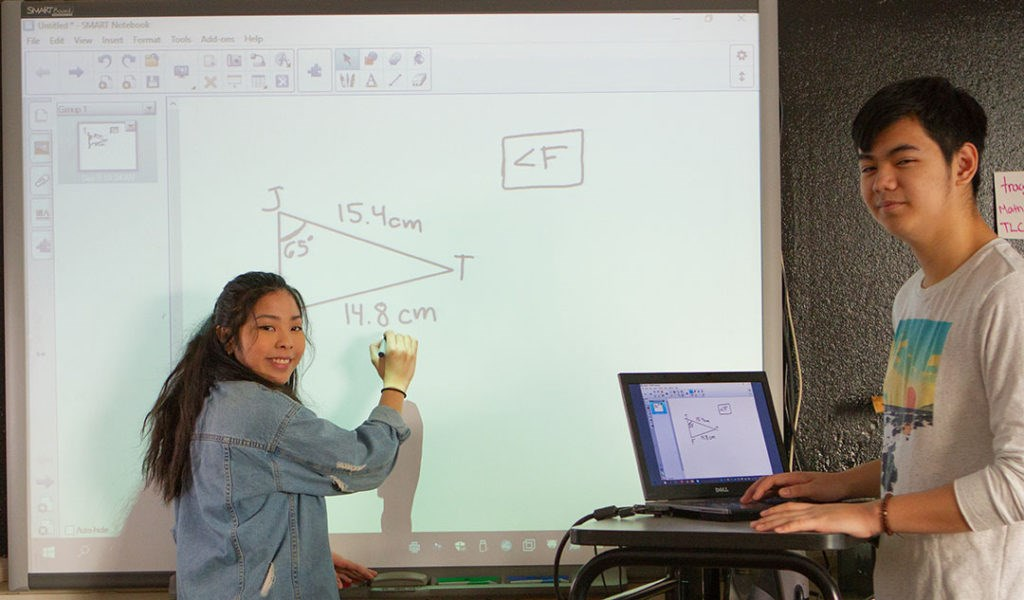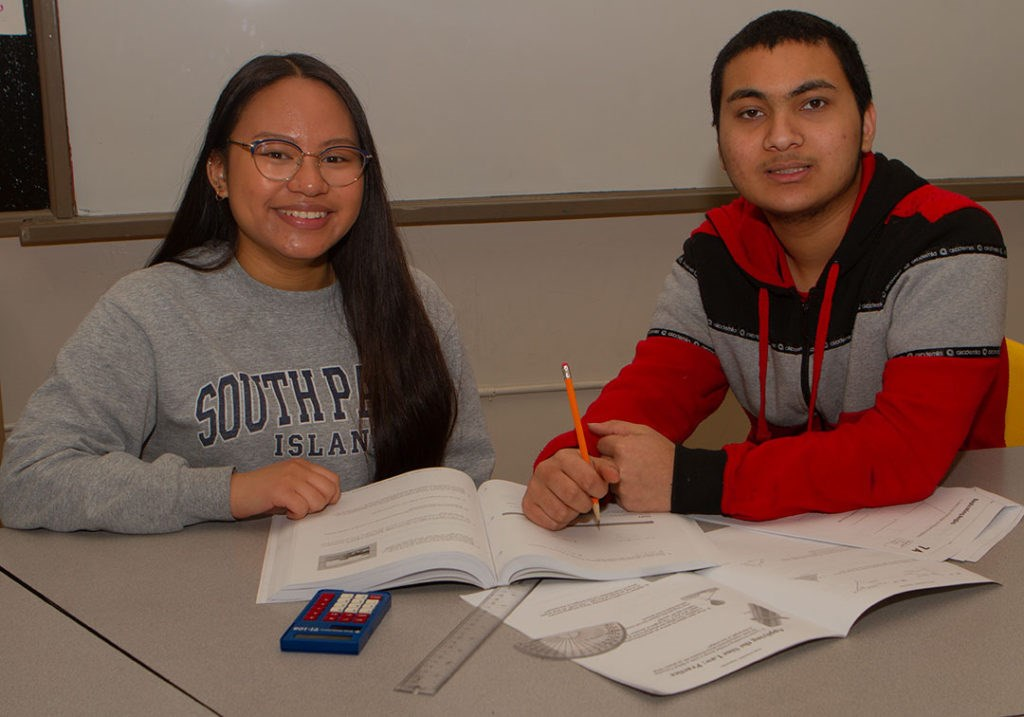Skip to main content

# Mathematics

April 14, 2021

Students need at least one math credit at each grade level for graduation. Students that have a high interest in Mathematics are encouraged to either take more than one math course at a particular level or take enriched math or Grade 12 Calculus.

## Grade 9

Mathematics 10F (1 credit) MATR1F or Advanced Mathematics 10F (1credit) MATE1F
This course if offered every day for 2 semesters, divided into 4 terms. Topics include: square roots and surface area, circle geometry, linear equations and inequalities, linear relations, powers and exponents, polynomials, similarities and transformations and probability and statistics. After completing this last year of general mathematics instruction, students will take IAPR2S and/or ESMR2S.. Mathematics 10F is a prerequisite for any grade 10 Mathematics course.

## Grade 10Intro to Applied & Pre-Calculus Math 20S (1 Credit) IAPR2S
This course blends contextual and algebraic mathematics by engaging students in activities that include the use of technology, problem solving, mental mathematics, and theoretical mathematics. Topics include:  measurement, trigonometry, factors and products, roots and powers, relations and functions, linear functions, and systems of equations. After completing this course students are encouraged to continue with Pre-Calculus 30S and/or Applied Math 30S. The prerequisite for this course is Grade 9 Math. This is a full year course.

Essential Mathematics 20S (1 Credit) ESMR2S
This course emphasizes consumer applications, problem solving, decision making, and spatial sense. Students are expected to work both individually and in small groups on mathematical concepts and skills encountered in everyday life in a technological society. Unit topics include analysis of games and numbers, personal finance, measurement, 2-D geometry, trigonometry, consumer decisions, transformations, and angle construction. After completing this course, students are encouraged to continue with Essential Math 30S. The prerequisite for this course is Grade 9 Math.

## Grade 11Applied Math 30S (1 Credit) APMR3S
This course promotes the learning of numerical and geometrical problem-solving techniques as they relate to the world around us. Technology such as graphing calculators, spreadsheets, and other computer software will be used by students as an integral part of both learning and assessment in this course. Topics include quadratic functions, proofs, statistics, systems of inequalities, trigonometry, and scale representations. After completing this course students are encouraged to continue with Applied Math 40S or Essential Math 40S. The prerequisite for this course is Intro to Applied and Pre-Calculus Math 20S.

Essential Math 30S (1 Credit) ESMR3S
This course builds on the concepts taught in Essential Math 20S. Unit topics include financial math, 3-D geometry, trigonometry, rate of change, and scale representations. After completing this course, students are encouraged to continue with Essential Math 40S. The prerequisite for this course is Grade 10 Math.

Pre-Calculus Math 30S (1 Credit) PCMR3S
The course comprises a high-level study of theoretical mathematics with an emphasis on problem solving and mental mathematics. The topics include the study of algebra, quadratic functions, reciprocal functions, and trigonometry. After completing this course, students are encouraged to continue with Pre-Calculus 40S, Applied Math 40S or Essential Math 40S. The prerequisite for this course is Intro to Applied and Pre-Calculus Math 20S.

## Grade 12Applied Math 40S (1 Credit) APMR4S
A graphing approach to problem solving highlights this course, as such, a graphing calculator is required. Calculators are available for loan from the teacher if needed. This course includes the topics: financial mathematics, logical reasoning, probability, relations and functions, and design and measurement. The prerequisite for this course is Pre-Calculus 30S or Applied Math 30S.

Essential Math 40S (1 Credit) ESMR4S
Unit topics include analysis of games and numbers, vehicle finance, home finance, statistics, geometry, trigonometry, precision measurement, business finance, a career life project, and probability. The prerequisite for this course is Grade 11 Math.

Pre-Calculus Math 40S (1 Credit) PCMR4S
The topics include the study of transformations of functions, trigonometric functions and identities, permutations and combinations, binomial theorem, polynomial functions, exponents and logarithms, and radical/rational equations and functions. The prerequisite for this course is Pre-Calculus 30S.  This is a full year course.

Calculus Math 45S (1/2 Credit) CALR4S
This course introduces students to the study of Calculus. This course focuses on the basic concepts of limits, derivatives, and integration. Students planning to pursue post secondary education in business, technology, science, or engineering will find this course of particular interest. This course is accepted at most universities and colleges as a grade 12 option credit for entrance requirements. The prerequisite for this course is Pre-Calculus 40S.

Back to top